# . One mole of SO2 gas and 1.00 mol of O2 gas were confined at 1000...

. One mole of SO2 gas and 1.00 mol of O2 gas were confined at 1000 K in a 5.00 L flask. The gases reacted, and at equilibrium, 0.840 mol of SO3 gas had formed. Calculate the value of Kc and Kp.

2SO2 (g) + O2 (g) ↔ 2SO3 (g)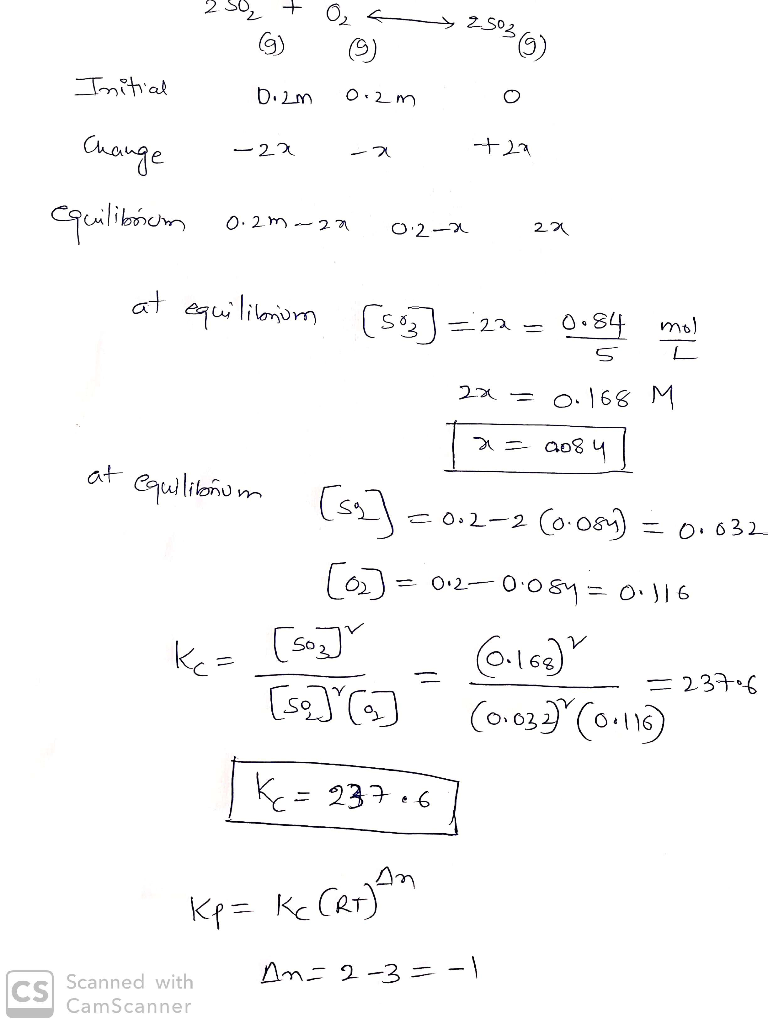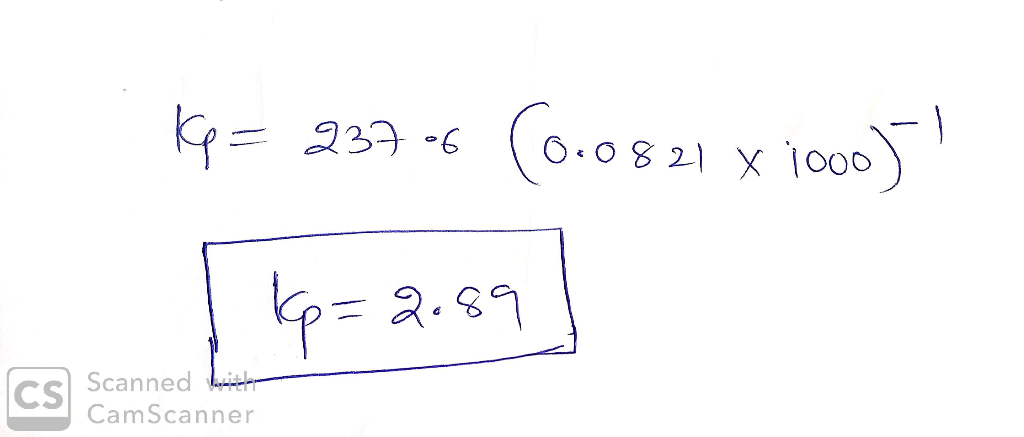#### Earn Coin

Coins can be redeemed for fabulous gifts.

Similar Homework Help Questions
• ### A 2.0 L flask is filled with 0.30 mol SO3 , 0.40 mol of SO2 and 0.50 mol of O2 and allowed to reach equilibrium. Assume...

A 2.0 L flask is filled with 0.30 mol SO3 , 0.40 mol of SO2 and 0.50 mol of O2 and allowed to reach equilibrium. Assume the temperature of the mixture is chosen so that Kc = 0.34. Predict the effect on the concentration of SO3 as the equilibrium is achieved by using Q, the reaction quotient. 2SO3(g) —> 2SO2(g) + O2(g)

• ### 1--N2(g) + O2(g)    2NO(g) is Kc = 4.1E-4. When a mixture of 0.30 mol of N2...1--N2(g) + O2(g)    2NO(g) is Kc = 4.1E-4. When a mixture of 0.30 mol of N2 and 0.30 mol O2 are allowed to react in a 2.0 L container, what is the equilibrium concentration of NO? 2--Consider the reaction CH4(g) + 2H2S(g)   CS2(g) + 4H2(g). Initially, 1.00 mol of CH4 and 1.00 mole of H2S are placed in a 250. mL container, and the system is allowed to reach equilibrium. If the equilibrium concentration of methane is 3.66 M, what...

• ### Consider the decomposition of sulfur trioxide gas into sulfur dioxide and oxygen 2SO3(g)<- ->2SO2(g) +O2(g) a...

Consider the decomposition of sulfur trioxide gas into sulfur dioxide and oxygen 2SO3(g)<- ->2SO2(g) +O2(g) a flask of SO3 is filled to 0.960 M and allowed to decompose. at equilibrium the concentration of SO2 is 0.320 M what is Kc?

• ### 1. 1 mole of SO2 and 1 mple of O2 are placed in a 1 liter...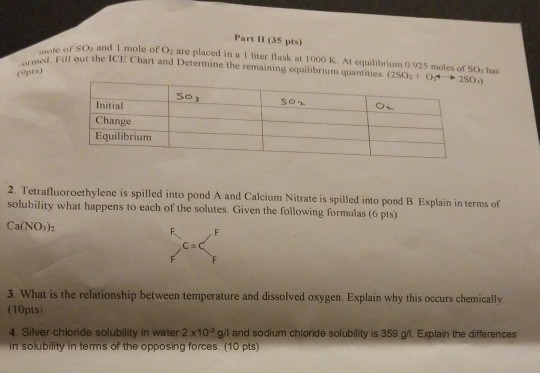1. 1 mole of SO2 and 1 mple of O2 are placed in a 1 liter flask at 1000K. At equilibrium 0.925 moles of SO3 has formed. Fill out the ICE Chart (provided in the picture) and Determine the remaining equilibrium quantities. (2SO2+O2 <-> 2SO3) 2. Tetrafluoroethylene is spilled into pond A and Calcium Nitrate is spilled into pond B. Explain in terms of solubility (include terms such as "like dissolves like", "polar", "nonpolar", "Ionic", and so on), what happens...

• ### For the equilibrium: 2 SO3(g) < = > O2(g) + 2 SO2(g) Kp = 0.269 at...

For the equilibrium: 2 SO3(g) < = > O2(g) + 2 SO2(g) Kp = 0.269 at 625 oC What is Kc at this temperature? Kp = Kc[RT]Δn R = 0.08206 L-atm/mol K

• ### At a certain temperature, 0.760 mol SO30.760 mol SO3 is placed in a 2.50 L2.50 L...

At a certain temperature, 0.760 mol SO30.760 mol SO3 is placed in a 2.50 L2.50 L container. 2SO3(g)−⇀↽−2SO2(g)+O2(g)2SO3(g)↽−−⇀2SO2(g)+O2(g) At equilibrium, 0.130 mol O20.130 mol O2 is present. Calculate Kc.

• ### 5. 2.00 mol each of SO2 and NO2 are introduced into a 1.00 L reactor at 300°C where Kp = 3.4. SO2(g) + NO2(g) <=>...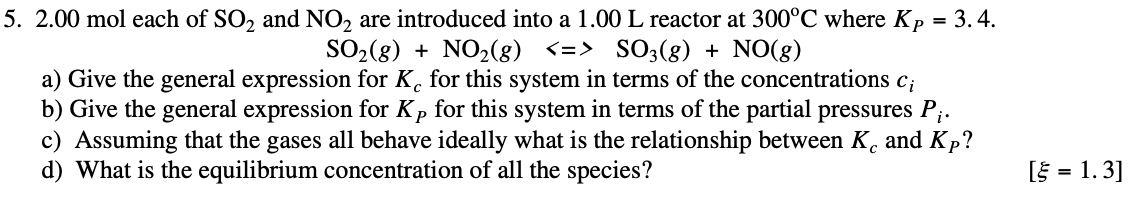5. 2.00 mol each of SO2 and NO2 are introduced into a 1.00 L reactor at 300°C where Kp = 3.4. SO2(g) + NO2(g) <=> SO3(g) + NO(g) a) Give the general expression for K, for this system in terms of the concentrations ci b) Give the general expression for Kp for this system in terms of the partial pressures Pi. c) Assuming that the gases all behave ideally what is the relationship between K, and Kp? d) What is...

• ### One step in the manufacture of sulfuric acid is the oxidation of SO2 gas to SO3:...

One step in the manufacture of sulfuric acid is the oxidation of SO2 gas to SO3: 2SO2 (g) + O2 (g) 2SO3 (g) ΔH = ‒196 kJ mol–1 This reaction is usually performed at 698 K and 1-2 atmospheres of pressure. 2. Use Le Chatelier’s principle to explain why decreasing the temperature of the reaction vessel to 298 K should theoretically increase the yield of SO3 (g)

• ### 2) For the equilibrium: 2 SO3(g) <=> O2(g) + 2 SO2(g) Kp = 0.269 at 625...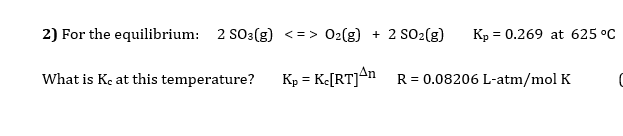2) For the equilibrium: 2 SO3(g) <=> O2(g) + 2 SO2(g) Kp = 0.269 at 625 °C What is Ke at this temperature? Kp = Kc[RT]An R = 0.08206 L-atm/mol K

• ### At 900 K the following reaction has Kp=0.345: 2SO2(g)+O2(g)???2SO3(g) In an equilibrium mixture the partial pressures...

At 900 K the following reaction has Kp=0.345: 2SO2(g)+O2(g)???2SO3(g) In an equilibrium mixture the partial pressures of SO2 and O2 are 0.150atm and 0.465atm , respectively. What is the equilibrium partial pressure of SO3 in the mixture?